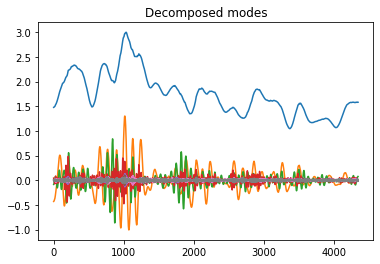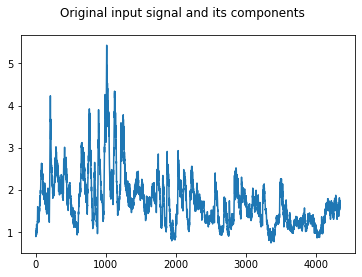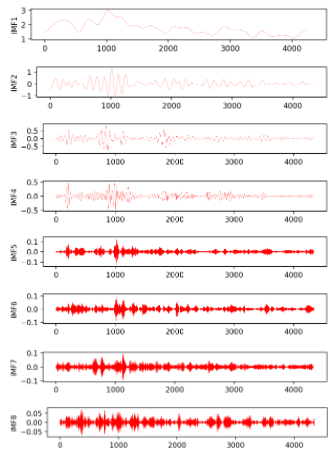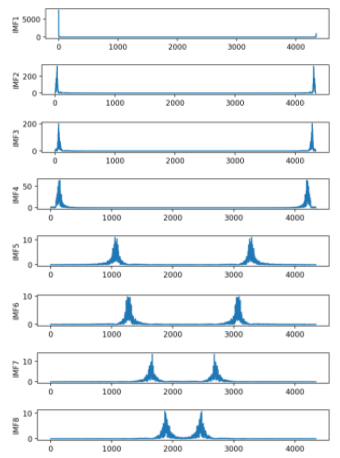# 数字信号处理技术（二）变分模态分解（VMD）-Python代码

VMD算法的介绍

## 2、 VMD应用实战

### 2.3 实验

``````import numpy as np
import matplotlib.pyplot as plt
from vmdpy import VMD
import pandas as pd
from scipy.fftpack import fft

filename= r'D:\研究生文件\代码区\2020.10.12\41046\41046ban.csv'

plt.plot(f.values)

alpha = 7000      # moderate bandwidth constraint
tau = 0.            # noise-tolerance (no strict fidelity enforcement)
K = 8        # 3 modes
DC = 0             # no DC part imposed
init = 1           # initialize omegas uniformly
tol = 1e-7

"""
alpha、tau、K、DC、init、tol 六个输入参数的无严格要求；
alpha 带宽限制 经验取值为 抽样点长度 1.5-2.0 倍；
tau 噪声容限 ；
K 分解模态（IMF）个数；
DC 合成信号若无常量，取值为 0；若含常量，则其取值为 1；
init 初始化 w 值，当初始化为 1 时，均匀分布产生的随机数；
tol 控制误差大小常量，决定精度与迭代次数
"""

u, u_hat, omega = VMD(f.values, alpha, tau, K, DC, init, tol)
plt.figure()

plt.plot(u.T)
plt.title('Decomposed modes')

fig1 = plt.figure()
plt.plot(f.values)

fig1.suptitle('Original input signal and its components')

for i in range(K):
plt.figure(figsize=(7,7), dpi=200)
plt.subplot(K,1,i+1)
plt.plot(u[i,:], linewidth=0.2, c='r')
plt.ylabel('IMF{}'.format(i+1))

# 中心模态
for i in range(K):
plt.figure(figsize=(7,7), dpi=200)
plt.subplot(K,1,i+1)
plt.plot(abs(fft(u[i,:])))
plt.ylabel('IMF{}'.format(i+1))

# 保存子序列数据到文件中
for i  in range(K):
a = u[i,:]
dataframe = pd.DataFrame({'v{}'.format(i+1):a})
dataframe.to_csv(r"D:\研究生文件\代码区\2020.10.12\41046\VMDban-%d.csv"%(i+1),index=False,sep=',')
``````

### 2.3 结果## 3. 总结

VMD可以很好的解决EMD 中出现的模态混叠现象，所以现在很多研究方向转向了VMD，利用其优点和其他的模型进行结合使用。
VMD的子序列个数的选择，有些是根据模态混叠现象，有些是进行迭代寻优，有些是自己指定个数等等方式。